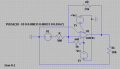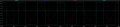# Determine the values of the resistances, which values should I use to solve it?

#### Javier Córdova

Joined Apr 17, 2021
2
Hello. I need conceptual help. I need to determine R, nR and mR in this circuit.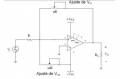I am using the following formulas to determine the values of the resistances: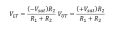I have:
Vsat =±13 V
RL=10kΩ
VLT = 2V
VUT = 4V

I am following the next procedure: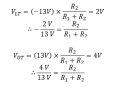I am having a real hard time determining which resistances to use in the procedure. I could really use some help, as I'm also struggling a lot with circuit analysis. (Does RL = R1?)

[Note: The problem suggest using a Potentiometer to adjust the resistance values, but I really don't have the knowledge, nor the resources. We are using LTSPICE XVII for these excercises]

I would really appreciate some help.This is what I've got, changing the resistance values manually: